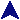Adder TestRuby Example Code#
# This tests the adder simulation by building various size adders and running
# them various numbers of times.
#
require "csim"

size = 8
rpt = 20
size = \$*.to_i if \$*.length > 0
rpt = \$*.to_i if \$*.length > 1

NumberOut.shush

# Blueprint for the 10-bit adder.

# Hook up two input switch banks, one output, and an overflow led.
na = SwitchBank.new
nb = SwitchBank.new
disp = NumberOut.new("  Sum")

#Gate.dump(na)

NumberOut.shush(false)

# Perform 30 addtions of random numbers.  For each, we choose two random
# inputs, and set them into the input switch banks.  The outputs will print,
# being the sum numeric display and the overflow LED.
print "== Performing #{rpt} tests on #{size}-bit adder.  ",
"Max sum is #{(1<<size)-1} ==\n"
rpt.times do
# Choose randoms and print them.
a = rand(1<<size)
b = rand(1<<size)
print a, " + ", b, ":\n"

# Add them, and keep the circuits active to suppress printout until after
# the whole operation is complete.
Gate.activate
na.value = a
nb.value = b
Gate.deactivate
end